# GA without Phenotype as Recursive Function

There are different possibilities how to describe the essential structures and properties of a genetic algorithm. Here we are looking to GAs as a recursive procedure.

Again we assume the maximal populationas a set of all possible strings of length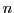over an alphabet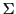as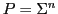, with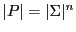. The working set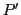is a subset ofas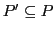. The fitness function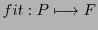maps all elements of the setinto a set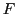of fitness values. We assume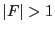, otherwise no discrimination would be possible (and then also no learning). We assume further that the 'goal' of an optimization process is not the 'complete' setbut some real subset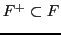where all values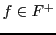are bigger than some threshold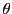. Complementary we have the set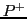as those elements ofwhose fitness values are all in the goal-set, written asor.

If we assume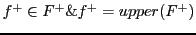as the maximal fitness value inand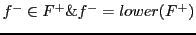as the lowest value inthen we can define a 'fuzzy' goal for a genetic process: with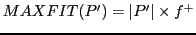andwe can define a 'goal region' like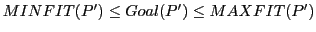.

With these definitions one can classify the history of of genetic process with terms like approaching or not approaching the goal. In both cases - approaching or not-approaching - one can introduce additional classifications like monotone or not-monotone. The non-monotone case can further be subdivided into periodic or a-periodic, which is the same as random.

Additionally to this overall categories one can measure the velocity of an approach based on the numbers of cycles used for approaching the goal state.

Depending of the resulting classification one can then investigate which properties of the genetic algorithm are responsible for this behavior.

In the literature one can find thoughts about the convergence of a series of populations generated by a GA (cf. e.g. Ankenbrandt 1991 , Rylander et.al 2001 , Langdon and Poli 2002 , and Fogel 2006 . Ankenbrandt presents the convergence problem in an algorithmic format (cf. :54)).

The main driver of a genetic process is the overall genetic function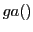. This function is a concatenated function. The most usual 'format' of a genetic functionis as follows: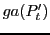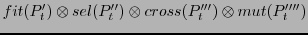(5.1)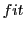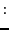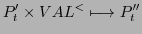(5.2)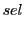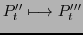(5.3)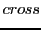(5.4)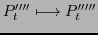(5.5)

Every genetic operator {fit, sel, cross, mut} occurs in the literature with many variants. Thus one has to give the explicit definitions every time one is using one of these variants.

Furthermore different configurations of these operators are possible. Some of these are listed here.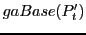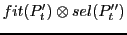(5.6)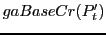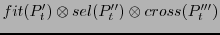(5.7)(5.8)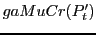(5.9)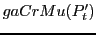(5.10)

If these different configurations have 'real' effects then one can measure these effects and one can compare the behavior directly. For these measurements one can use the terms introduced above.

Experimental Procedure

1.2. while(){
3.;
4. t++;
5. }
6.Subsections
Gerd Doeben-Henisch 2013-01-14